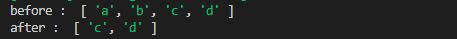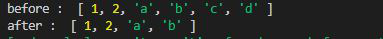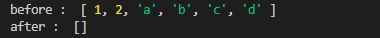# Lodash _.drop() Method

• Last Updated : 02 Feb, 2022

Lodash is a JavaScript library that works on the top of underscore.js. Lodash helps in working with arrays, strings, objects, numbers etc.
The Lodash.drop() method is used to drop the elements in a given array.

Syntax :

`Lodash.drop(array, number)`

Parameters:

• Array: It is the original array from which the elements are to be removed.
• Number: It is the number of elements to be removed from the array.

Note: The elements are removed from the index 0 of the array.

Return Value: It returns the sliced array.

Example 1:

## Javascript

 `// Requiring the lodash library` `const _ = require(``"lodash"``);`   `// Original array` `let array = [``"a"``, ``"b"``, ``"c"``, ``"d"``]`   `// using drop() method to remove` `// first two elements` `let newArray = _.drop(array, 2)`   `// Printing original array ` `console.log(``"before : "``, array)`   `// Printing array after applying` `// drop function` `console.log(``"after : "``, newArray)`

Output:Example 2: If elements to be removed from the right side of the array we use _.dropRight() function.

## Javascript

 `// Requiring the lodash library` `let lodash = require(``"lodash"``);`   `// Original array` `let array = [1, 2, ``"a"``, ``"b"``, ``"c"``, ``"d"``]`   `// Using drop() method to remove ` `// first 2 elements from right` `let newArray = lodash.dropRight(array, 2)`   `// Printing original array ` `console.log(``"before : "``, array)`   `// Printing array after applying` `// drop function` `console.log(``"after : "``, newArray)`

Output:Example 3: If number greater then the size of array is given then it returns the empty array as given in the below given example.

## Javascript

 `// Requiring the lodash library` `let lodash = require(``"lodash"``);`   `// Original array` `let array = [1, 2, ``"a"``, ``"b"``, ``"c"``, ``"d"``]`   `// Using drop() method to remove` `// first 10 elements` `let newArray = lodash.drop(array, 10)`   `// Printing original array ` `console.log(``"before : "``, array)`   `// Printing array after applying` `// drop function` `console.log(``"after : "``, newArray)`

Output:My Personal Notes arrow_drop_up
Recommended Articles
Page :